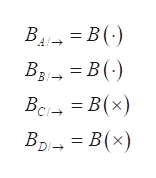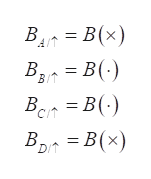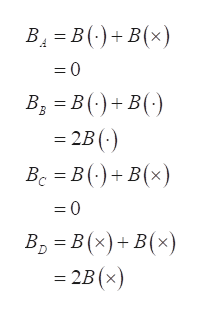Question
36 views

Two long, straight wires cross each other at right angles, and
each carries the same current as in Figure CQ19.18. Which
of the following statements are true regarding the total
magnetic field at the various points due to the two wires?
(There may be more than one correct statement.) (a) The
field is strongest at points B and D. (b) The field is strongest
at points A and C. (c) The field is out of the page at point B
and into the page at point D. (d) The field is out of the page
at point C and out of the page at point D. (e) The field has
the same magnitude at all four points.

check_circle

Step 1

Let all the points be equidistant from both the wires.

As the current in both the wires is same, the individual magnitude of magnetic field at all the points due to each wire will be same and will be equal to B.

Due to the current carrying conductor in right direction, the field at point A and B will be outside the page, and at point C and D will be inside the page, according to right hand thumb rule.

Write the notations for the magnetic field.

Here right arrow shows the field due to horizontal wire, dot shows the direction as outside the page and the cross shows the direction as inside the page.help_outlineImage Transcriptionclose= B (·) BAI- Вз- 3DВ() BI→ Вс — В(х) Вр — В(х) B(: Bo B, DI- fullscreen
Step 2

Due to the current carrying conductor in upward direction, the field at point B and C will be outside the page, and at point A and D will be inside the page, according to right hand thumb rule.

Write the notations for the magnetic field.

Here upward arrow shows the field due to vertical wire, dot shows the direction as outside the page and the cross shows the direction as inside the page.help_outlineImage TranscriptioncloseB = B(x) = B(-) BIT Bct Bar = B(-) В 3 В(х) DIT fullscreen
Step 3

Write the net magnetic fiel...help_outlineImage TranscriptioncloseВ — В()+ в(x) B3 = B (·)+ B(· = 2B (:) Вс — В () + B(х) В, — В (%) + B(х) = 2B(x) fullscreen

### Want to see the full answer?

See Solution

#### Want to see this answer and more?

Solutions are written by subject experts who are available 24/7. Questions are typically answered within 1 hour.*

See Solution
*Response times may vary by subject and question.
Tagged in

### Magnetic Effects of Current